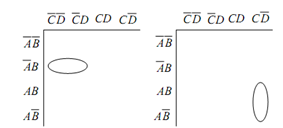## Karnaugh Simplifications Assignment Help

Assignment Help: >> Karnaugh Map and Combinatorial Logic Design - Karnaugh Simplifications

Karnaugh Simplifications and Simplified Logic Circuits:

Pairs

There are two possible pairs in K-maps: Horizontally adjacent 1s pair (encircled 1s in Figure (a)) and vertically adjacent 1s pair (encircled 1s in Figure (b)). The SOP equation for the Figure (a) may be simplified as

Y = A¯ BC¯ D¯ +  A¯ BC¯ D = A¯ BC¯ (D¯ + D) = A¯ BC¯

It implies that as we move from the first basic product (AB C D) to the second one (A¯B C¯ D¯), only one variable (D for this case) modify from complemented to un-complemented form and that variable shall get removed since D¯ + D = 1 is one of the Boolean identities. Likewise, for vertically adjacent 1s pairs in Figure (b), one variable (B for this case) shall be eliminated. Therefore a pair leads to removal of one variable and their complements.(a)                                              (b)

Figure: (a) Horizontally Adjacent 1s Pair; and (b) Vertically Adjacent 1s Pair in K-maps